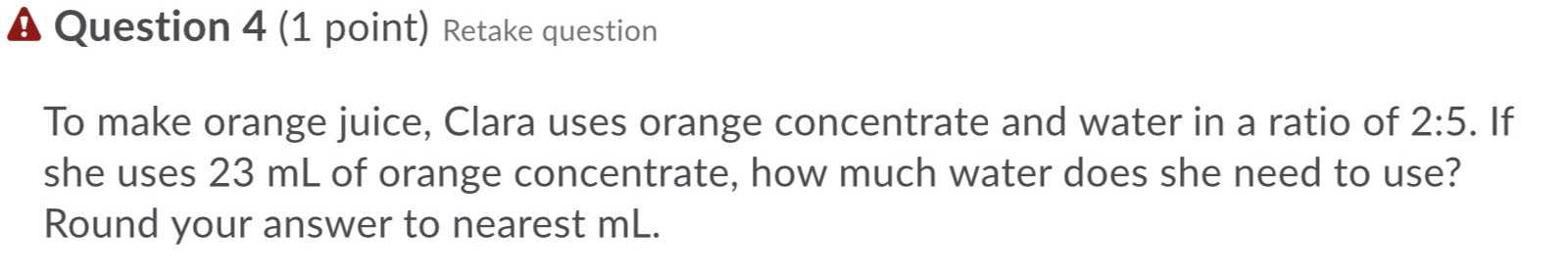### ¿Todavía tienes preguntas de matemáticas?

Pregunte a nuestros tutores expertos
Algebra
PreguntaA Question $$4$$ (1 point) To make orange juice, Clara uses orange concentrate and water in a ratio of $$2 : 5$$ . If she uses $$23 mL$$ of orange concentrate, how much water does she need to use? Round your answer to nearest mL.

ratio of orange concentrate and water = 2 : 5

quantity of orange concentrate = 23 ml

let quantity of water = x

$$\frac{2}{5}= \frac{23}{x}$$

x = 57.5

quantity of water = 57.5 ml

Solución
View full explanation on CameraMath App.